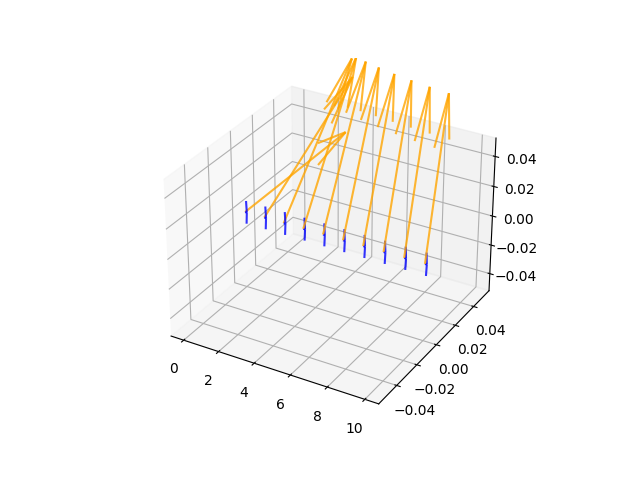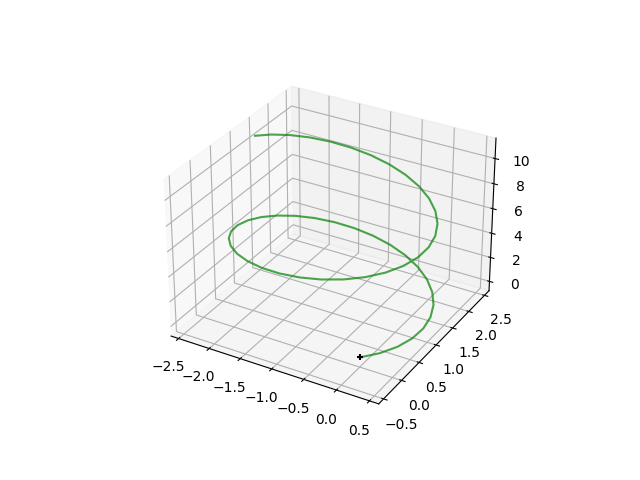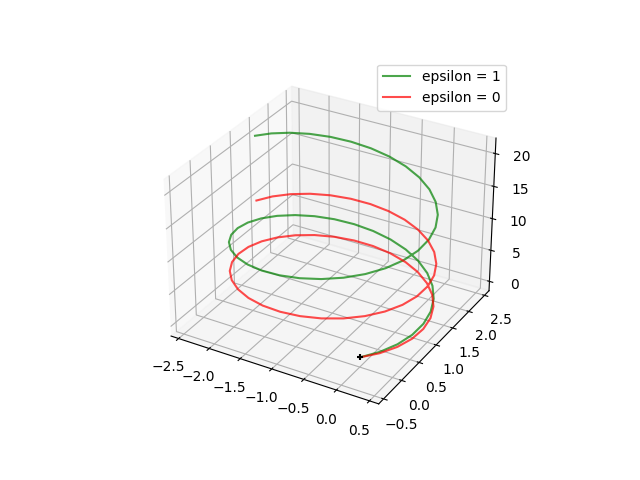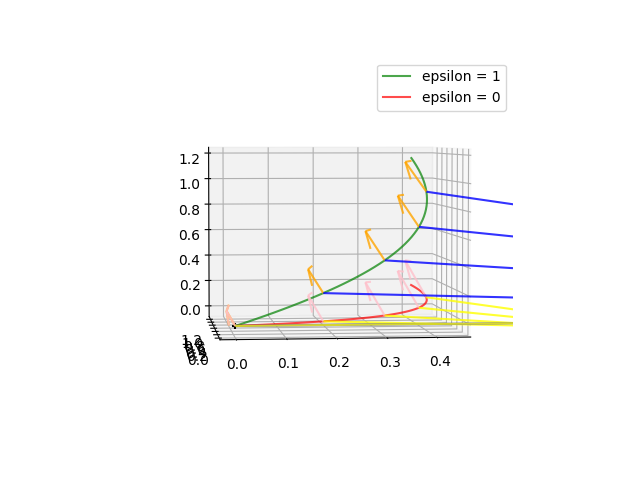# Tutorial: Sub-Riemannian geometry on the Heisenberg group#

 In :

import geomstats.backend as gs
import geomstats

from geomstats.geometry.sub_riemannian_metric import SubRiemannianMetric
from geomstats.geometry.heisenberg import HeisenbergVectors

%matplotlib notebook

INFO: Using autograd backend


We import modules for plotting,

 In :

import matplotlib.pyplot as plt
import matplotlib
from mpl_toolkits.mplot3d import Axes3D


## The Heisenberg group#

We instantiate the 3D heisenberg group,

 In :

heis = HeisenbergVectors()


‘heis’ is an object representing a manifold, without any sub-Riemannian structure associated to it.

In geomstats, a sub-Riemannian structure is constructed via the SubRiemannianMetric-class. We now instantiate a SubRiemannianMetric corresponding to the heisenberg group.

In geomstats, a sub-Riemannian metric can be instantiated by supplying either

• a function which at an input point outputs the matrix representing the cometric, or

• a function which at an input point outputs a matrix whose columns are frame-vectors spanning the distribution at that point. This frame is then implicitly assumed to be orthonormal, so it determines both the distribution and the metric on the tangent bundle.

## Defining the Heisenberg sub-Riemannian structure via a frame-field#

We will construct the sub-Riemannian Heisenberg group by supplying a frame-function. Mathematically, we represent the distribution $$\Delta$$ in a standard way; at the point $$p \in heis$$ the distribution is

$\Delta_p = \text{span}(F_X(p), F_Y(p)),$

where $$F_X = L^{\star}_p(X)$$, $$F_Y = L^{\star}_p(Y)$$ and $$X = (1,0,0)$$, $$Y=(0,1,0)$$. So e.g. $$F_X$$ is the tangent left translation to point $$p$$ of the standard basis vector X in the Lie-algebra. In geomstats, this can be done concisely as follows,

 In :

def heis_frame(point):
translations = heis.jacobian_translation(point)
return translations[..., 0:2]


Given the frame, we now instantiate the sub-Riemannian metric for the Heisenberg group

 In :

heis_sr = SubRiemannianMetric(dim=3, frame=heis_frame)


We can illustrate the distribution by plotting the frame-vectors along a line:

 In :

line = gs.array([k*gs.array([1., 1., 1.]) for k in gs.linspace(0,10,10)])
line = gs.array([k*gs.array([1., 0., 0.]) for k in gs.linspace(0,10,10)])

quiver_length = 0.1

fig1 = plt.figure()
ax  = plt.axes(projection='3d')

for i in range(line.shape):
point = line[i,:]
frame_at_point = heis_sr.frame(point)

frame1 = frame_at_point[:, 1]
frame2 = frame_at_point[:, 0]

ax.quiver(point, point, point,
frame1, frame1, frame1,
length = quiver_length, normalize = True, color = 'orange',alpha=0.8,
linestyle = '-')
ax.quiver(point, point, point,
frame2, frame2, frame2,
length = quiver_length, normalize = True, color = 'blue',alpha=0.8,
linestyle = '-')Everything is now set up to generate sub-Riemannian geodesics. A sub-Riemannian geodesic on a manifold $$M$$ is determined by an initial point $$p \in M$$ and an initial cotangent $$\lambda \in T_p M$$.

 In :

base_point = gs.array([0., 0., 0.])
initial_cotangent = gs.array([1., 1., 1.])

times = gs.linspace(0., 10., 50)

path = heis_sr.geodesic(initial_point=base_point,
initial_cotangent_vec=initial_cotangent,
n_steps=1000)

path_1_1 = path(times)

 In :

fig1 = plt.figure()
ax = plt.axes(projection='3d')
ax.scatter(0, 0, 0, marker='+', color='black')

ax.plot3D(path_1_1[:, 0],
path_1_1[:, 1],
path_1_1[:, 2],
'-',
linewidth=1.5,
markersize=0,
marker='.',
color='green',
alpha=0.7)

plt.show()## Sub-Riemannian structures as limits of Riemannian structures#

If the distribution $$\Delta$$ is of the same dimension as the manifold at every point, the structure is actually Riemannian. In geomtats, if the manifold is $$n$$-dimensional and we pass a frame function with $$n$$ linearly independent vectors at every point, this will generate the Riemannian structure with a metric that makes these vectors orthonormal at every point.

For the heisenberg group, we can use the frame given by left-translation of the standard basis in the Lie algebra,

$\{F_X(p), F_Y(p), \epsilon\cdot F_Z(p)\},$

where $$\epsilon \in [0,1]$$. For $$\epsilon=0$$ the geodesics will be those of the standard Heisenberg group. For $$\epsilon = 1$$ the geodesics will be those of the left-invariant Riemannian metric which on the algebra is simply the euclidean metric.

 In :

def heis_frame_riemannian(point, epsilon):
frame_matrix = heis.jacobian_translation(point)
scale_matrix = gs.array([gs.ones((3,)), gs.ones((3,)), gs.ones((3,))*epsilon]).T
return  gs.einsum('...ij,...ij -> ...ij', frame_matrix, scale_matrix)

heis_epsilon_1 = SubRiemannianMetric(dim=3, frame=lambda point : heis_frame_riemannian(point, 1))
heis_epsilon_0 = SubRiemannianMetric(dim=3, frame=lambda point : heis_frame_riemannian(point, 0))

 In :

base_point = gs.array([0., 0., 0.])
initial_cotangent = gs.array([1., 1., 1.])

times = gs.linspace(0., 10., 50)

path_epsilon_1 = heis_epsilon_1.geodesic(initial_point=base_point,
initial_cotangent_vec=initial_cotangent,
n_steps=1000)

path_epsilon_0 = heis_epsilon_0.geodesic(initial_point=base_point,
initial_cotangent_vec=initial_cotangent,
n_steps=1000)

path_1 = path_epsilon_1(times)
path_0 = path_epsilon_0(times)

fig1 = plt.figure()
ax = plt.axes(projection='3d')
ax.scatter(0, 0, 0, marker='+', color='black')

ax.plot3D(path_1[:, 0],
path_1[:, 1],
path_1[:, 2],
'-',
linewidth=1.5,
markersize=0,
marker='.',
color='green',
alpha=0.7,
label='epsilon = 1')

ax.plot3D(path_0[:, 0],
path_0[:, 1],
path_0[:, 2],
'-',
linewidth=1.5,
markersize=0,
marker='.',
color='red',
alpha=0.7,
label='epsilon = 0')

ax.legend()

plt.show()We see that the spiralling structure is similar for the Riemannian ($$\epsilon=1$$) and the sub-Riemannian ($$\epsilon=0$$) geodesics, in fact only their z-coordinates differ. Let’s check that the sub-Riemannian geodesic is horizontal wrt. to the distribution $$\Delta_p = \text{span}(F_X(p), F_Y(p))$$ (i.e. it’s tangent vectors lies in $$\Delta$$), whereas the Riemannian one isn’t.

 In :

base_point = gs.array([0., 0., 0.])
initial_cotangent = gs.array([1., 1., 1.])

times = gs.linspace(0., 1., 50)

path_epsilon_1 = heis_epsilon_1.geodesic(initial_point=base_point,
initial_cotangent_vec=initial_cotangent)

path_epsilon_0 = heis_epsilon_0.geodesic(initial_point=base_point,
initial_cotangent_vec=initial_cotangent)

path_1 = path_epsilon_1(times)
path_0 = path_epsilon_0(times)

fig1 = plt.figure()
ax = plt.axes(projection='3d')
ax.scatter(0, 0, 0, marker='+', color='black')

ax.plot3D(path_1[:, 0],
path_1[:, 1],
path_1[:, 2],
'-',
linewidth=1.5,
markersize=0,
marker='.',
color='green',
alpha=0.7,
label='epsilon = 1')

ax.plot3D(path_0[:, 0],
path_0[:, 1],
path_0[:, 2],
'-',
linewidth=1.5,
markersize=0,
marker='.',
color='red',
alpha=0.7,
label='epsilon = 0')

ax.legend()

quiver_length = 1

exp_curve = path_1

for i in range(exp_curve.shape):
if i % 10 == 0:
point = exp_curve[i,:]
frame_at_point = heis_sr.frame(point)

frame1 = frame_at_point[:, 1]
frame2 = frame_at_point[:, 0]

ax.quiver(point, point, point,
frame1, frame1, frame1,
length = quiver_length, normalize = True, color = 'orange',alpha=0.8,
linestyle = '-')
ax.quiver(point, point, point,
frame2, frame2, frame2,
length = quiver_length, normalize = True, color = 'blue',alpha=0.8,
linestyle = '-')

quiver_length = 1

exp_curve = path_0

for i in range(exp_curve.shape):
if i % 10 == 0:

point = exp_curve[i,:]
frame_at_point = heis_sr.frame(point)

frame1 = frame_at_point[:, 1]
frame2 = frame_at_point[:, 0]

ax.quiver(point, point, point,
frame1, frame1, frame1,
length = quiver_length, normalize = True, color = 'pink',alpha=0.8,
linestyle = '-')
ax.quiver(point, point, point,
frame2, frame2, frame2,
length = quiver_length, normalize = True, color = 'yellow',alpha=0.8,
linestyle = '-')

ax.view_init(3, -96)

plt.show()